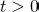# Using hazard ratios to estimate causal effects in RCTs

Odd Aalen and colleagues have recently published an interesting paper on the use of Cox models for estimating treatment effects in randomised controlled trials. In a randomised trial we have the treatment assignment variable X, and an often used primary analysis is to fit a simple Cox model with X as the only covariate. This gives an estimated hazard ratio comparing the hazard in the treatment group compared to the control, and this is assumed constant over time. In any trial, there will almost certainly exist other variables Z, some of which might be measured, and some of which will always be unmeasured, and which influence the outcome. At baseline, X and Z are statistically independent as a result of randomisation, which of course is the reason randomisation in general allows us to make a causal statement about the treatment effect – we need not worry about confounding.

More hazards of hazard ratios
The first part of their paper shows that in general X and Z are no longer independent if one looks only at individuals who are still event free at some time. This is the case unless the true hazard function is additive in X and Z. They then note that this additive property is not satisfied by the Cox model which includes X and Z as covariates. Moreover, they note that if in truth the hazard was additive in X and Z, this would not imply a Cox model conditional only on X, i.e. the simple Cox model that simply includes treatment group as the only covariate, and which is very often used as the primary analysis of an RCT with a time to event outcome.

The fact that the two groups are no longer balanced in respect of covariates Z at timeswas noted before by Aalen himself, and also by others such as Miguel Hernán in the context of interpreting of changing hazard ratios in observational studies (the hazard of hazard ratios). Because at later times the groups are no longer balanced with respect to these covariates, direct contrasts of hazards at timesare subject to confounding, despite the fact that treatment was randomly assigned.

Aalen et al also explain that because of these results, the hazard ratio for treatment does not have a causal interpretation. This applies even if the Cox model fits the observed data well. That is, even if the Cox model with X as covariate fits well, i.e. the observed hazard function in the two groups are proportional, the estimated hazard ratio cannot be given a clear interpretation in terms of a causal effect for treatment.

Alternatives
What then could one do instead, in order to estimate a well defined causal effect in an RCT with time to event outcome? Aalen et al suggest the use of accelerated failure time models or other models based on transforming the event times, in which one is modelling the actual distribution of survival times, rather than the hazard function.

In their draft book on causal inference, Miguel Hernán and James Robins also discuss the problem of estimating causal effects with survival data (Chapter 17). They emphasize that an attractive alternative is to consider the binary variable ‘survival to time x’, where x is a suitably chosen time. By doing this, one returns to the simple situation of a binary outcome, and the only complication is handling censoring in the estimation process.

Competing risks
However, matters are more complex if the event of interest (e.g. occurrence of some disease, or death due to a particular cause) need not necessarily occur for all subjects. This can often be the case because an individual may die prior to the event of interest occurring (or may die of a cause other than the one of interest). Here death can be considered a competing event. In such cases it is unclear how one can unambiguously define a meaningful outcome variable for each individual, in order to define a causal effect. In their causal inference book, Hernán and Robins consider a number of possible strategies that one might take to handle the competing event (Fine point 17.1), but conclude:

None of these strategies solves the problem of truncation by death satisfactorily. Truncation by competing events raises logical questions about the meaning of the causal estimand that cannot be bypassed by statistical techniques.

It would thus seem that there is more work to do in order to define causal effects in such cases!

This site uses Akismet to reduce spam. Learn how your comment data is processed.